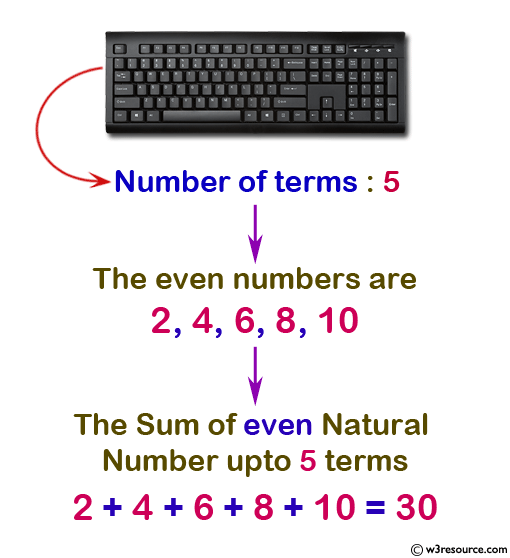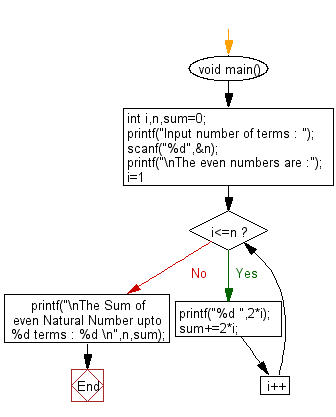﻿ C : Calculate n terms of even natural number and their sum

# C Exercises: Calculate n terms of even natural number and their sum

## C For Loop: Exercise-16 with Solution

Write a C program to display the sum of n terms of even natural numbers.

Visual Presentation:Sample Solution:

C Code:

``````#include <stdio.h>  // Include the standard input/output header file.

int main() {
int i, n, sum = 0;  // Declare variables 'i' for loop counter, 'n' for user input, 'sum' to store the sum.

printf("Input number of terms : ");  // Prompt the user to input the number of terms.
scanf("%d", &n);  // Read the value of 'n' from the user.

if (n <= 0) {
printf("Number of terms should be a positive integer.\n");
return 1;  // Exit the program with an error code.
}

printf("\nThe even numbers are :");  // Print a message.

for (i = 1; i <= n; i++) {  // Start a loop to generate even numbers.
int evenNumber = 2 * i;  // Calculate the even number.
printf("%d ", evenNumber);  // Print the even number.
sum += evenNumber;  // Add the even number to the sum.
}

printf("\nThe Sum of even Natural Number up to %d terms : %d\n", n, sum);  // Print the sum of even numbers.

return 0;  // Return 0 to indicate successful execution.
}

```
```

Sample Output:

```Input number of terms : 5

The even numbers are :2 4 6 8 10
The Sum of even Natural Number upto 5 terms : 30
```

Flowchart:C Programming Code Editor:

What is the difficulty level of this exercise?

Test your Programming skills with w3resource's quiz.

﻿